### Introduction to Machine Learning -- evaulating chemical composition of wine

We will walk through an example that involves training a model to tell what kind of wine will be "good" or "bad" based on a training set of wine chemical characteristics.

First, we're going to import the packages that we'll be using throughout this notebook. Then we'll bring in the CSV from my desktop. You can get the raw data from UCI's ML Database.

We're also using sci-kit learn. For more information on installing sci-kit to use sklearn packages, visit this website.

In:
```#Importing required packages.
import pandas as pd
import matplotlib.pyplot as plt
import seaborn as sb
import numpy as np
%matplotlib inline

#Importing sklearn packages
from sklearn.svm import SVC, LinearSVC
from sklearn.linear_model import SGDClassifier
from sklearn.metrics import confusion_matrix, classification_report
from sklearn.preprocessing import StandardScaler, LabelEncoder
from sklearn.model_selection import train_test_split, GridSearchCV, cross_val_score, StratifiedKFold
from sklearn.linear_model import LogisticRegression
from sklearn.neighbors import KNeighborsClassifier
from sklearn.naive_bayes import GaussianNB
from sklearn.metrics import accuracy_score
```
In:
```#import data and view first few rows of in a dataframe

#Let's preview the dataframe
```
Out:
fixed
acidity
volatile
acidity
citric
acid
residual
sugar
chlorides free sulfur
dioxide
total sulfur
dioxide
density pH sulphates alcohol quality
0 7.4 0.70 0.00 1.9 0.076 11.0 34.0 0.9978 3.51 0.56 9.4 5
1 7.8 0.88 0.00 2.6 0.098 25.0 67.0 0.9968 3.20 0.68 9.8 5
2 7.8 0.76 0.04 2.3 0.092 15.0 54.0 0.9970 3.26 0.65 9.8 5
3 11.2 0.28 0.56 1.9 0.075 17.0 60.0 0.9980 3.16 0.58 9.8 6
4 7.4 0.70 0.00 1.9 0.076 11.0 34.0 0.9978 3.51 0.56 9.4 5

Now that we have the data in a format that's usable for us within a Jupyter notebook, let's take a closer look at the data.
There's a lot of numbers... and that's great! It's not necessary that we understand the intricacy of all of the numbers and the meaning being the measurements for the purpose of this exercise. If it makes you feel more comfortable, feel free to look at this link. Kaggle provides a great breakdown for each of the variables.

However, it's important that we identify what will be inputs for our model and what will be the factor we're trying to determine. In this scenario, our goal is to determine whether the wine is "good" or "bad". We'll use the "quality" field to determine "good" or "bad". The rest of the variables in the table will be inputs for our model.

Next step: Understanding the variable statistics

For us to be able to use this data confidently, we need to ensure the data we're using is clean and is not missing many variables. Let's take a quick crack at ensuring that the data meets these two conditions.

In:
```#checking the number of records imported
len(red_wine_df.index)
```
Out:
`1599`
In:
```#running descriptive statistics across all the variables
red_wine_df.describe()
```
Out:
fixed
acidity
volatile
acidity
citric
acid
residual
sugar
chlorides free sulfur
dioxide
total sulfur
dioxide
density pH sulphate alcohol quality
count 1599.00 1599.00 1599.00 1599.00 1599.00 1599.00 1599.00 1599.00 1599.00 1599.00 1599.00 1599.00
mean 8.319637 0.527821 0.270976 2.538806 0.087467 15.874922 46.467792 0.996747 3.311113 0.658149 10.422983 5.636023
std 1.741096 0.179060 0.194801 1.409928 0.047065 10.460157 32.895324 0.001887 0.154386 0.169507 1.065668 0.807569
min 4.600000 0.120000 0.000000 0.900000 0.012000 1.000000 6.000000 0.990070 2.740000 0.330000 8.400000 3.000000
25% 7.100000 0.390000 0.090000 1.900000 0.070000 7.000000 22.000000 0.995600 3.210000 0.550000 9.500000 5.000000
50% 7.900000 0.520000 0.260000 2.200000 0.079000 14.000000 38.000000 0.996750 3.310000 0.620000 10.200000 6.000000
75% 9.200000 0.640000 0.420000 2.600000 0.090000 21.000000 62.000000 0.997835 3.400000 0.730000 11.100000 6.000000
max 15.900000 1.580000 1.000000 15.500000 0.611000 72.000000 289.000000 1.003690 4.010000 2.000000 14.900000 8.000000

In:
```#checking to see if there's any null variables
red_wine_df.isnull().sum()
```
Out:
```fixed acidity           0
volatile acidity        0
citric acid             0
residual sugar          0
chlorides               0
free sulfur dioxide     0
total sulfur dioxide    0
density                 0
pH                      0
sulphates               0
alcohol                 0
quality                 0
dtype: int64```

In:
```# listing the unique values for the wine quality
red_wine_df['quality'].unique()
```
Out:
`array([5, 6, 7, 4, 8, 3])`

In:
```#taking a look at the quality ranges
sb.countplot(x='quality', data=red_wine_df)
```
Out:In:
```# generating charts that compare all of the variables
# against quality, although not necessary, it's good to understand the data
df1 = red_wine_df.select_dtypes([np.int, np.float])

for i, col in enumerate(df1.columns):
plt.figure(i)
sb.barplot(x='quality', y =col, data=df1)
```
Out: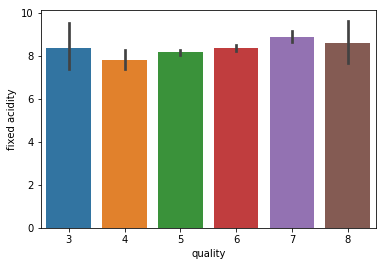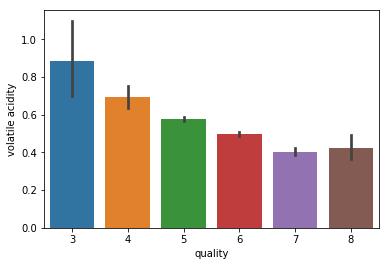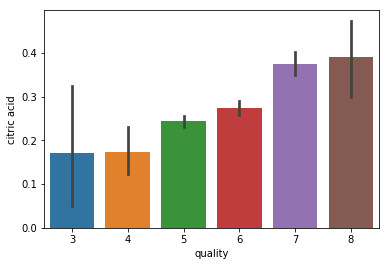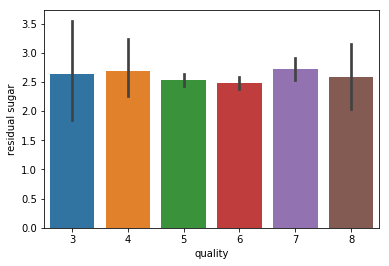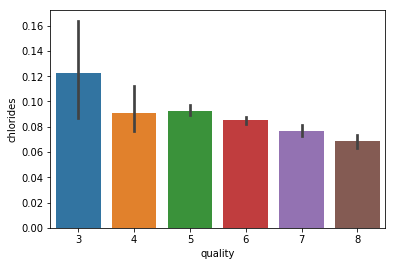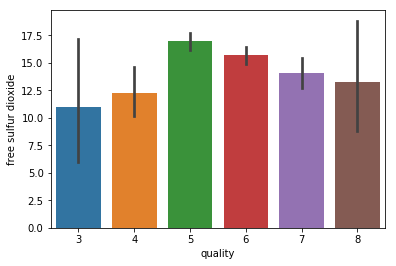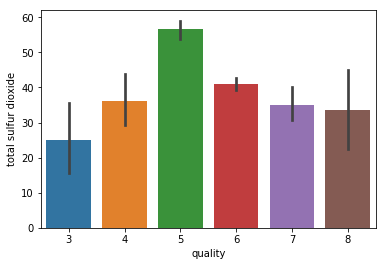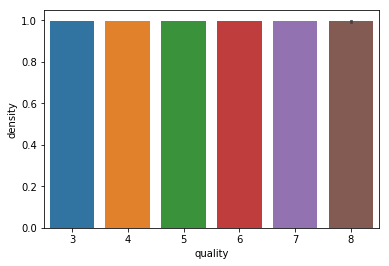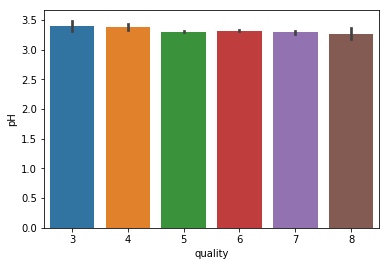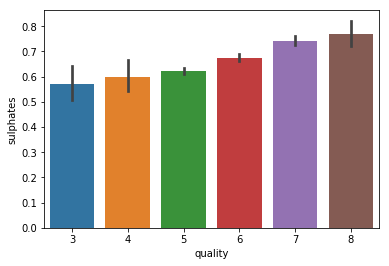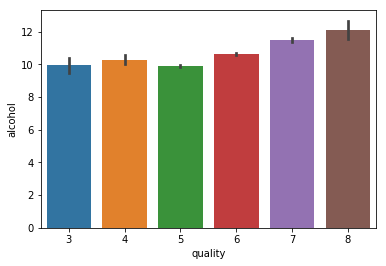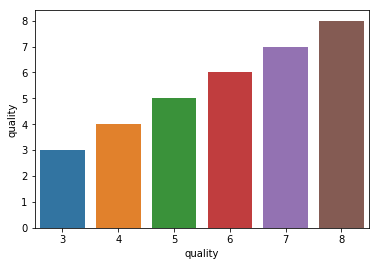Preprocessing data before for modeling

Now that we know our data is pretty clean, and we have a good idea of what our data looks like, we're going pre-processing the data before plugging these variables into the model.

What we need to do:

1. Need to split the "quality" column into "good" and "bad", and assign numeric values for good and bad
2. We need to split out training and testing data.

1. Spliting the quality column into "good" and "bad"

In:
```# splitting wine into good and bad groups, we're saying here that wines that have a quality score between
# 2-6.5 are "bad" quality, and wines that are  between 6.5 - 8 are "good"
bins = (2, 6.5, 8)
red_wine_df['quality'] = pd.cut(red_wine_df['quality'], bins = bins, labels = group_names)
```
In:
```# however "bad" and "good" aren't good naming conventions for a model to read in, so we're going to
# assign a numeric label for this value. LabelEncoder() will help us do this!

# Assigning a label to our quality variable
label_quality = LabelEncoder()

# Now changing our dataframe to reflect our new label
red_wine_df['quality'] = label_quality.fit_transform(red_wine_df['quality'])
```
In:
```# printing the head to ensure the transformation happened
```
Out:
fixed
acidity
volatile
acidity
citric
acid
residual
sugar
chlorides free sulfur
dioxide
total sulfur
dioxide
density pH sulphates alcohol quality
0 7.4 0.70 0.00 1.9 0.076 11.0 34.0 0.9978 3.51 0.56 9.4 0
1 7.8 0.88 0.00 2.6 0.098 25.0 67.0 0.9968 3.20 0.68 9.8 0
2 7.8 0.76 0.04 2.3 0.092 15.0 54.0 0.9970 3.26 0.65 9.8 0
3 11.2 0.28 0.56 1.9 0.075 17.0 60.0 0.9980 3.16 0.58 9.8 0
4 7.4 0.70 0.00 1.9 0.076 11.0 34.0 0.9978 3.51 0.56 9.4 0

2. Splitting out training data and testing data

First we'll split out the labels and the features, then we will split the testing and training data out.

In:
```# extracting all model inputs from the data set
all_inputs = red_wine_df[['fixed acidity', 'volatile acidity', 'citric acid', 'residual sugar',
'chlorides', 'free sulfur dioxide', 'total sulfur dioxide', 'density',
'pH', 'sulphates', 'alcohol']].values
# extracting quality labels
all_labels = red_wine_df['quality'].values
# a test to see what the inputs look like
all_inputs[:2]
```
Out:
```array([[ 7.4   ,  0.7   ,  0.    ,  1.9   ,  0.076 , 11.    , 34.    ,
0.9978,  3.51  ,  0.56  ,  9.4   ],
[ 7.8   ,  0.88  ,  0.    ,  2.6   ,  0.098 , 25.    , 67.    ,
0.9968,  3.2   ,  0.68  ,  9.8   ]])```
In:
```# Next we will apply standard scaling
# standard scaling allows us to normalize all of the data such
# that the distribution will have a mean value of 0 and a standard deviation of 1
# this step is useful when we want to compare data that corresponds to different units
sc = StandardScaler()
all_inputs = sc.fit_transform(all_inputs)
```
In:
```# the function, train_test_split, will take our inputs and labels and split them out into training
# and testing subsections for us
# test_size parameter = proprotion of data that should be kept aside for testing, in this case 1/4 of the data
# random_state = theseed used by the random number generator
(training_inputs,
testing_inputs,
training_classes,
testing_classes) = train_test_split(all_inputs, all_labels, test_size=0.25, random_state=1)
```

Modeling!

We're going to use a decision tree classifier to begin with, let's see how it works for us!

In:
```#trying decision tree classfier
from sklearn.tree import DecisionTreeClassifier

# Create the classifier
decision_tree_classifier = DecisionTreeClassifier()

# Train the classifier on the training set
decision_tree_classifier.fit(training_inputs, training_classes)

# Validate the classifier on the testing set using classification accuracy
decision_tree_classifier.score(testing_inputs, testing_classes)
```
Out:
`0.855`

The decision tree classifer's wasn't terrible. We're going to try experimenting with a few different classifers, then once we pick one we'll dig into optimizing it.

In:
```#selecting the models and the model names in an array
models=[LogisticRegression(),
LinearSVC(),
SVC(kernel='rbf'),
KNeighborsClassifier(),
RandomForestClassifier(),
DecisionTreeClassifier(),
GaussianNB()]
model_names=['Logistic Regression',
'Linear SVM',
'rbf SVM',
'K-Nearest Neighbors',
'Random Forest Classifier',
'Decision Tree',
'Gaussian NB']

# creating an accuracy array and a matrix to join the accuracy of the models
# and the name of the models so we can read the results easier
acc=[]
m={}

# next we're going to iterate through the models, and get the accuracy for each
for model in range(len(models)):
clf=models[model]
clf.fit(training_inputs,training_classes)
pred=clf.predict(testing_inputs)
acc.append(accuracy_score(pred,testing_classes))

m={'Algorithm':model_names,'Accuracy':acc}

# just putting the matrix into a data frame and listing out the results
acc_frame=pd.DataFrame(m)
acc_frame
```
Out:
Algorithm Accuracy
0 Logistic Regression 0.8850
1 Linear SVM 0.8925
2 rbf SVM 0.9000
3 K-Nearest Neighbors 0.8750
4 Random Forest Classifier 0.9075
5 Decision Tree 0.8800
7 Gaussian NB 0.8250

Based on this single run, it looks like the Random Forest performed the best. Let's try to optimize it a bit more.

We're going to use a grid search test different parameter changes within the RandomForestClassifer, cross validates each one and determines which combintation provides the best performance.

In:
```random_forest_classifier = RandomForestClassifier()

# setting up the parameters for our grid search
# You can check out what each of these parameters mean on the Scikit webiste!
# http://scikit-learn.org/stable/modules/generated/sklearn.ensemble.RandomForestClassifier.html
parameter_grid = {'n_estimators': [10, 25, 50, 100, 200],
'max_features': ['auto', 'sqrt', 'log2'],
'criterion': ['gini', 'entropy'],
'max_features': [1, 2, 3, 4]}

# Stratified K-Folds cross-validator allows us mix up the given test/train data per run
# with k-folds each test set should not overlap across all shuffles. This allows us to
# ultimately have "more" test data for our model
cross_validation = StratifiedKFold(n_splits=10)

# running the grid search function with our random_forest_classifer, our parameter grid
# defineda bove, and our cross validation method
grid_search = GridSearchCV(random_forest_classifier,
param_grid=parameter_grid,
cv=cross_validation)

# using the defined grid search above, we're going to test it out on our
# data set
grid_search.fit(all_inputs, all_labels)

# printing the best scores, parameters, and estimator for our Random Forest classifer
print('Best score: {}'.format(grid_search.best_score_))
print('Best parameters: {}'.format(grid_search.best_params_))

grid_search.best_estimator_
```
Out:
```Best score: 0.8830519074421513  Best parameters: {'criterion': 'entropy', 'max_features': 2, 'n_estimators': 50}
```
```RandomForestClassifier(bootstrap=True, class_weight=None, criterion='entropy',
max_depth=None, max_features=2, max_leaf_nodes=None,
min_impurity_decrease=0.0, min_impurity_split=None,
min_samples_leaf=1, min_samples_split=2,
min_weight_fraction_leaf=0.0, n_estimators=50, n_jobs=1,
oob_score=False, random_state=None, verbose=0,
warm_start=False)```

In:
```#Now we can take the best classifier from the Grid Search and use that for our classifer
random_forest_classifier = grid_search.best_estimator_

rf_df = pd.DataFrame({'accuracy': cross_val_score(random_forest_classifier, all_inputs, all_labels, cv=10),
'classifier': ['Random Forest'] * 10})
rf_df.mean()
```
Out:
```accuracy    0.874939
dtype: float64```

In:
```#plotting our accuracy results!!
sb.boxplot(x='classifier', y='accuracy', data=rf_df)
sb.stripplot(x='classifier', y='accuracy', data=rf_df, jitter=True, color='black')
```
Out: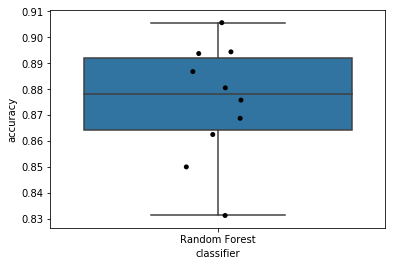Our classifer on average, has an accuracy of 87.6%, not too bad! If you want more material on this data set, check out Kaggle for additional examples!